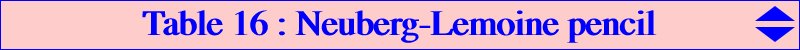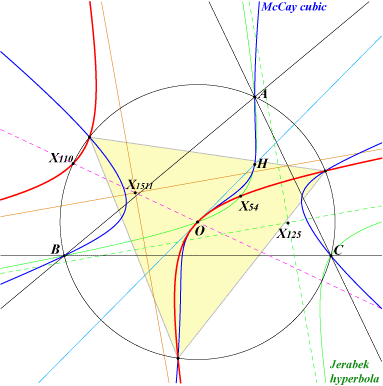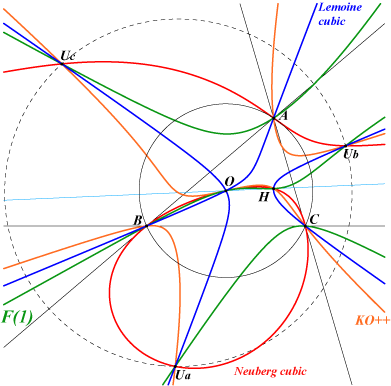The Neuberg cubic K001 and the Lemoine cubic K009 generate a pencil of cubics passing through A, B, C, O, H and the three points Ua, Ub, Uc mentioned in the Neuberg cubic page. These cubics have the same tangent at O which is the line O-X(54). See a generalization in table 18. Each cubic of this pencil meets the Euler line at O, H and a third point X such that OX = t OH (vectors), in which case the cubic is denoted by F(t). Special cases : F(0) = K009 (Lemoine cubic) : the only nodal cubic with node O. F(infinity) = K001 (Neuberg cubic) : the only circular cubic. F(-1) = K080 (KO++) : the only equilateral cubic, the only K+ cubic. Properties : F(t) meets the circumcircle at the same points as pK(X6, X). F(t) meets the line at infinity at the same points as pK(X6, Y) where Y is defined by XY = OH (vectors). The isogonal conjugate Y* of Y lies on F(t). For any t different of -1, the locus of points whose polar conic is a rectangular hyperbola is the Euler line. Hence the points O, H, X are the only points on F(t) with such polar conic. When t = -1, the polar conic of any point of the plane is a rectangular hyperbola since F(-1) is a K60. The locus of points whose polar conic is a circle is the rectangular hyperbola (H) passing through O, X(54), X(110), X(182), X(1147), X(1385), the vertices of the circumnormal triangle. Its asymptotes are parallel to those of the Jerabek hyperbola. Its center is X(1151), the midpoint of O-X(110). The tangent at O is the Euler line.F(t) meets the sidelines of ABC again at U, V, W. The triangles ABC and UVW are perspective if and only if t = 0 (U, V, W are the midpoints of ABC), t = infinity (U, V, W are the traces of X(30), the infinite point of the Euler line) or t = -1/3 (U, V, W are the traces of the isotomic conjugate of X(381), the midpoint of GH). Connection with CL055 : Any cubic F(t) is spK(Y, M) as in CL055 with X, Y as above and M midpoint of XY. The isogonal transform F(t)* of F(t) is then spK(X, M) passing through O, H, Ua*, Ub*, Uc*and meeting the line at infinity like pK(X6, X), the circumcircle like pK(X6, Y). See also Table 54. *** The following table gives a selection of cubics from the Neuberg-Lemoine pencil.X t Y F(t) or X(i) on F(t) pK(X6, Y) with same asymptotic directions pK(X6, X) with same points on the circumcircle F(t)* X(30) infty X(30) K001 Neuberg K001 K001 K001 X(3) 0 X(4) K009 Lemoine K006 Orthocubic K003 McCay K028 X(20) -1 X(3) K080 KO++ K003 McCay K004 Darboux K405 X(4) 1 X(382) X(3), X(4) pK(X6, X382) K006 Orthocubic X(2) 1/3 X(3830) X(2), X(3), X(4), X(3830)* pK(X6, X3830) K002 Thomson X(5) 1/2 X(3627) X(3), X(4), X(5), X(3627)* pK(X6, X3627) K005 Napoleon X(3534) -2/3 X(2) X(3), X(4), X(6), X(3627)* K002 Thomson X(550) -1/2 X(5) X(3), X(4), X(54), X(550) K005 Napoleon pK(X6, X550) X(376) -1/3 X(381) X(3), X(4), X(376) pK(X6, X381) K243 X(64)* -2 X(20) X(3), X(4), X(64), X(1657) K004 DarbouxThe figure below shows several interesting cubics.